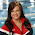## 5.19.2014

### Measuring Battery Life -- BeagleBone Black

As part of our float we want to be able to constantly check the battery life of our submersible. To do this we wanted to be able to use the BeagleBone. Because the ADC (Analog Digital Converter) on the BBB (BeagleBone Black) has a max voltage of 1.8v, we needed a way of dropping the 24v battery. To do this we used a voltage divider. By using the voltage divider to step-down the 24v to <1.8v we are able to accurately measure voltage by using a simple ratio. With this measured voltage we can make sure that our 24v LiFePO4 batteries never drop below 20v (the voltage is each cell should be 3.2v when fully charged and 2.5 when empty)

First, we needed to calculate the Vout using the voltage divider formula:

We needed a voltage <1.8v and therefore used a 47 ohm and a 1k ohm resistor to drop the 25.6v to approximately 1.15v.

Vout = 25.6(47/1047)
Vout = 1.15

By measuring the voltage across R2 (from Vout to Ground) you will see a voltage of around 1.15v

Next you need to use Ohm's Law and Joule's Law to calculate the power consumption of the resistors. Using V=IR and Wattage=I^2R you should find the power consumption of R1 to be a little over .5W (as a shortcut you can use this calculator).

Our setup uses 2W resistors and still gets a little hot, although this is expected while using resistors and high powered devices. Also make sure that R2 is less than 1K ohms as that will interfere with the analog readings.

To measure the voltage with the BeagleBone we used the ADC capabilities Adafruit's BBIO Library. By residing the voltage periodically and then comparing it to a pre-determined ratio we're able to accurately measure the battery life. An image of the code is below:

If you would like to download the code we used find it at our GitHub Page

#### 1 comment:

1.When it comes to a battery it is important to charge it complete. Using the specific voltage and current charger which are provided by the company of which brand you are using. batteroo